# Dividing Fractions, Whole Numbers, and Mixed Numbers Lesson Plan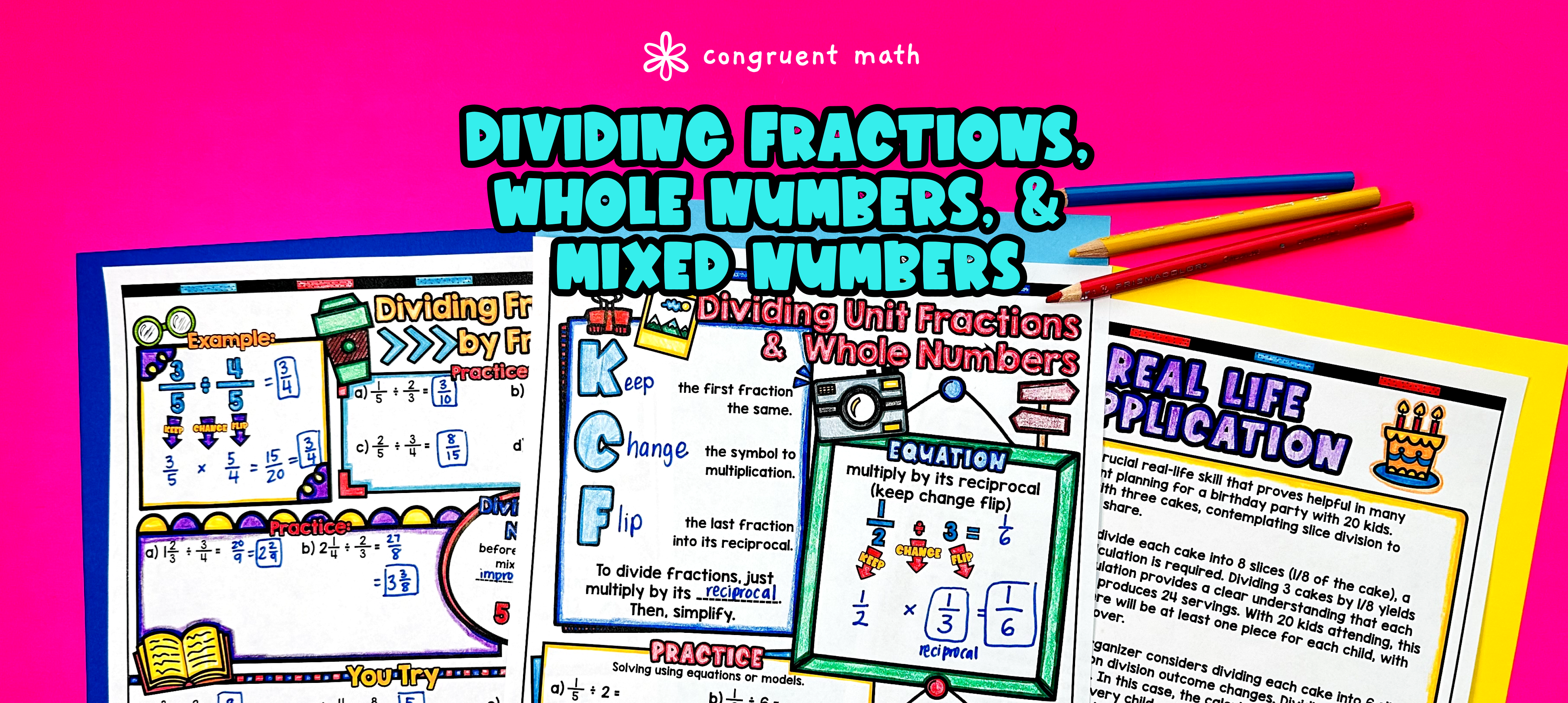Pin This

## Overview

Ever wondered how to teach dividing fractions, whole numbers, and mixed numbers in an engaging way to your 5th and 6th-grade students?

In this lesson plan, students will learn about fraction division and its real-life applications. Through artistic and interactive guided notes, check for understanding activities, a doodle & color by number activity, and a maze worksheet, students will gain a comprehensive understanding of dividing fractions, whole numbers, and mixed numbers.

The lesson culminates with a real-life example that explores how fraction division can be used in practical situations. This lesson plan provides a structured and engaging way for students to learn and practice dividing fractions, whole numbers, and mixed numbers.

## Get the Lesson Materials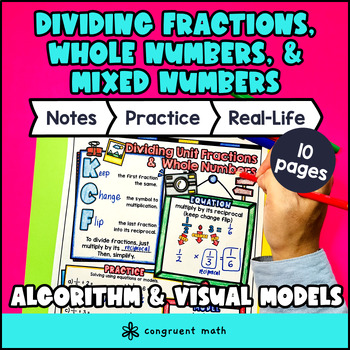\$4.25

## Learning Objectives

After this lesson, students will be able to:

• Divide fractions by whole numbers

• Divide fractions by unit fractions

• Divide fractions by mixed numbers

• Apply the concept of dividing fractions to real-life math problems

## Prerequisites

Before this lesson, students should be familiar with:

• Basic multiplication and division skills, including dividing whole numbers by whole numbers and multiplying fractions by whole numbers.

• Understanding of the concept of fractions, including how to represent fractions on a number line and compare fractions.

• Basic understanding of mixed numbers, including how to convert mixed numbers to improper fractions and vice versa.

## Key Vocabulary

• Dividing fractions

• Whole numbers

• Unit fractions

• Mixed numbers

## Procedure

### Introduction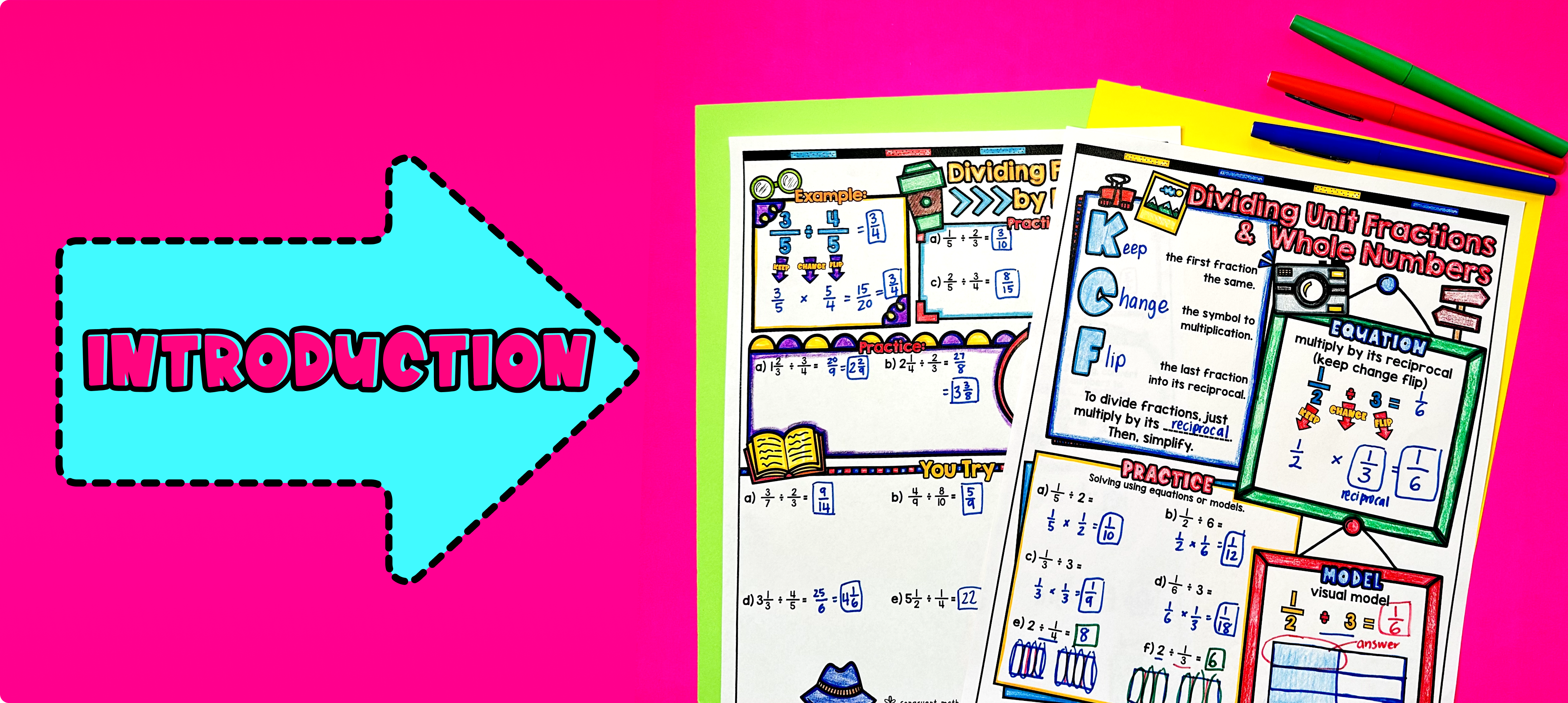Pin This

As a hook, ask students why dividing fractions is important in real life situations. Refer to the last page of the guided notes as well as the FAQs below for ideas.

Use the first page of the guided notes to introduce the concept of dividing fractions by whole numbers. Walk through the key points of dividing fractions by a whole number, such as dividing the numerator by the whole number and keeping the denominator the same. Refer to the FAQ below for a walk through on this, as well as ideas on how to respond to common student questions.

Use the second page of the guided notes to introduce how to divide fractions by mixed numbers. Based on student responses, reteach the concept of dividing fractions to those who need extra help. If your class has a wide range of proficiency levels, you can pull out students for reteaching, and have more advanced students begin work on the practice exercises.

### Practice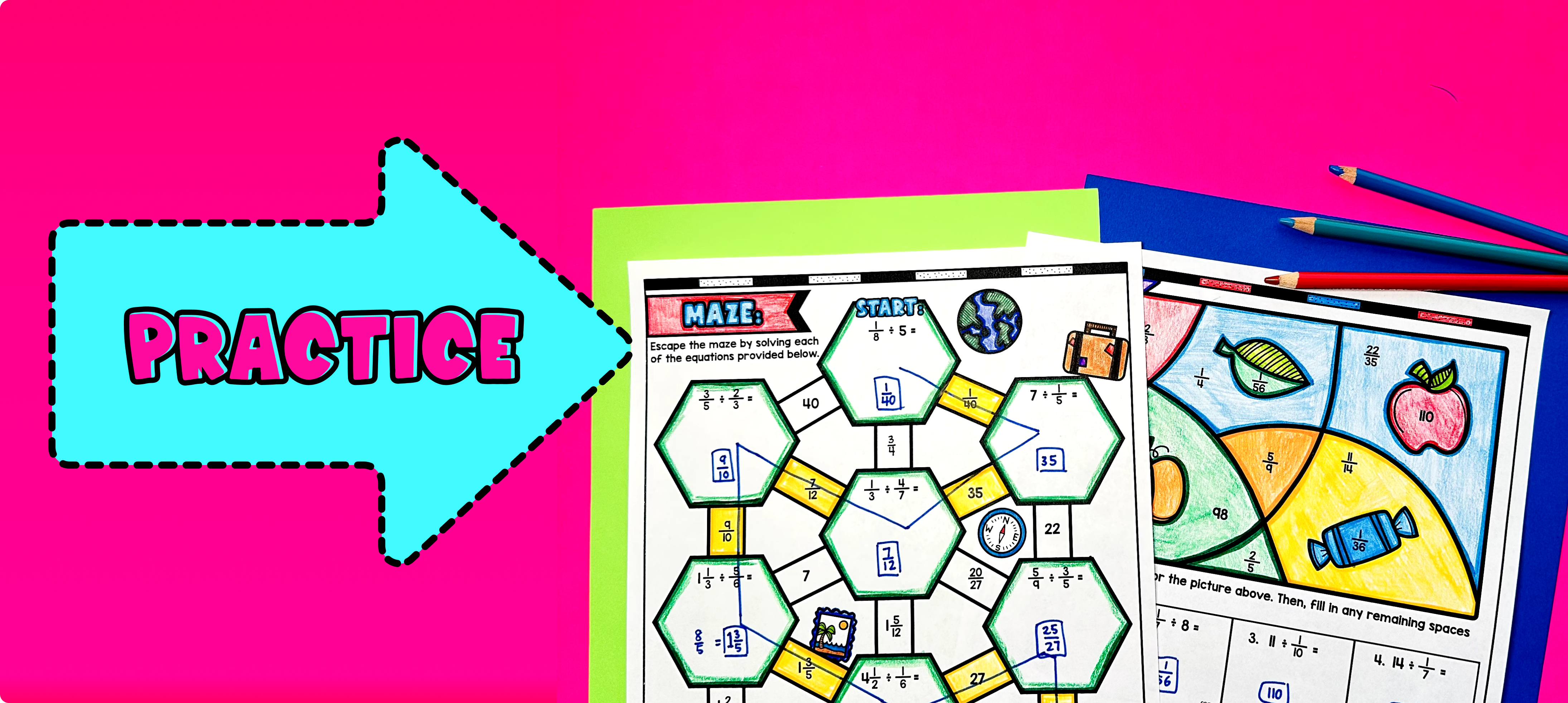Pin This

Have students practice dividing fractions, whole numbers, and mixed numbers using the coloring worksheet activity. Walk around to answer student questions.

Fast finishers can dive into the maze activity for extra practice. You can assign it as homework for the remainder of the class.

### Real-Life Application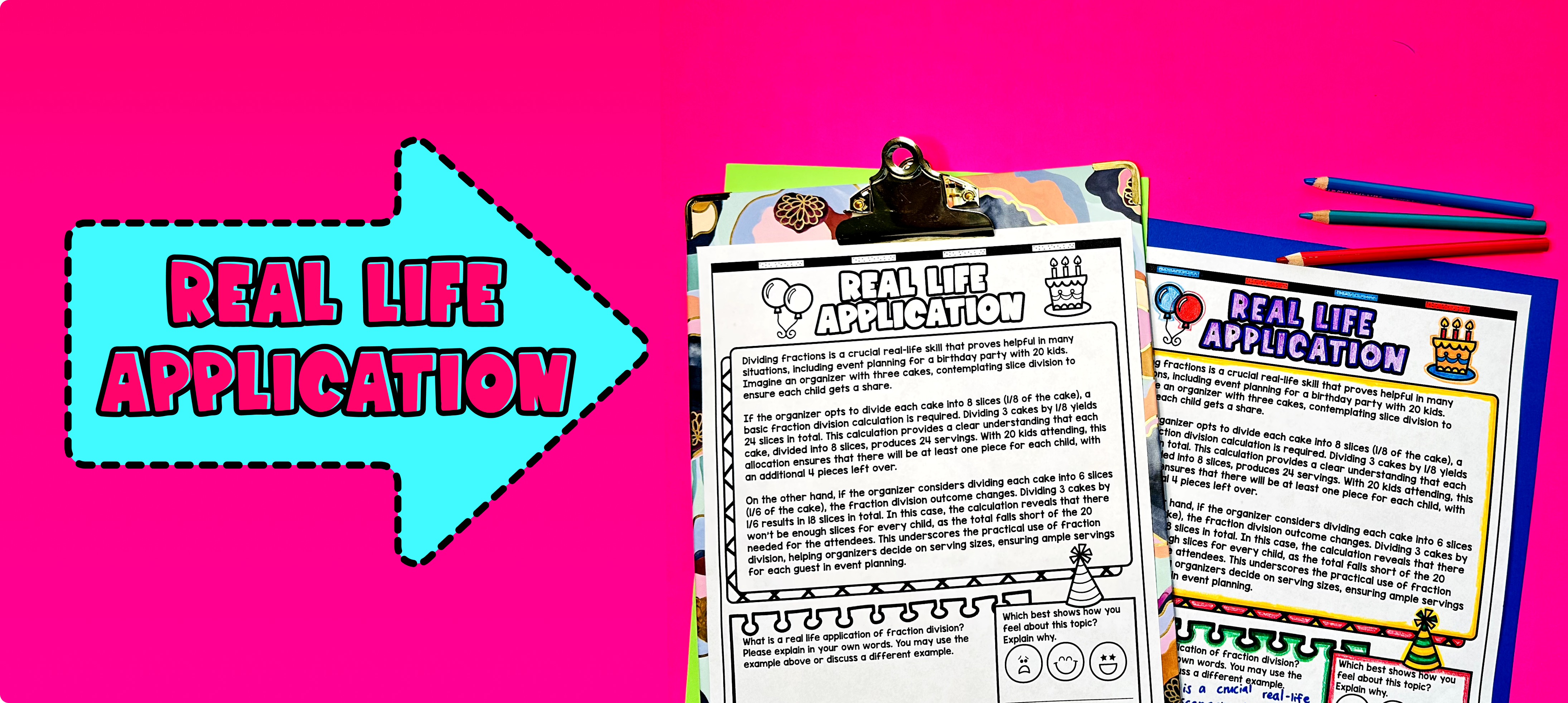Pin This

Bring the class back together, and introduce the concept of real-life applications of dividing fractions, whole numbers, and mixed numbers. Explain to the students that understanding how to divide fractions is not only useful in solving math problems but also in real-world situations.

Give examples of real-life scenarios where dividing fractions, whole numbers, and mixed numbers would be necessary. Some examples could include:

1. Recipes: Explain that in baking and cooking, recipes often require using fractions. For example, if a recipe calls for 1/2 cup of flour and you want to make half of the recipe, you would need to divide the amount of flour by 2 to get 1/4 cup.

2. Measurement: Discuss how dividing fractions can be used when splitting a whole into equal parts. For instance, if a group of friends wants to equally share 3/4 of a pizza, they would need to divide the pizza into 4 equal parts and each person would get 3/16 of the pizza.

Encourage students to brainstorm other real-world situations where dividing fractions, whole numbers, and mixed numbers may be used. Refer to the FAQ section for more ideas on how to teach real-life applications of dividing fractions.